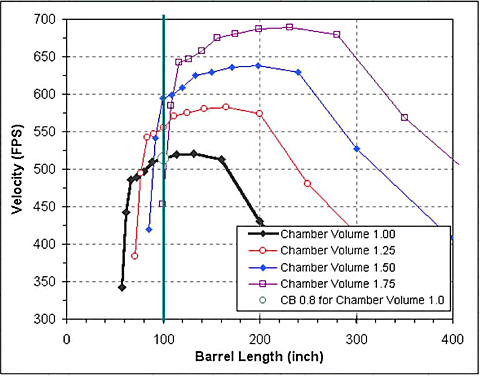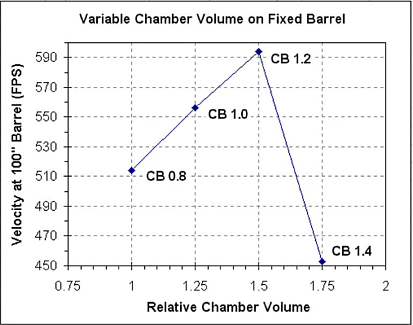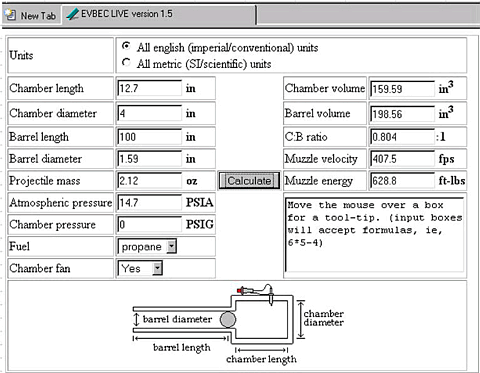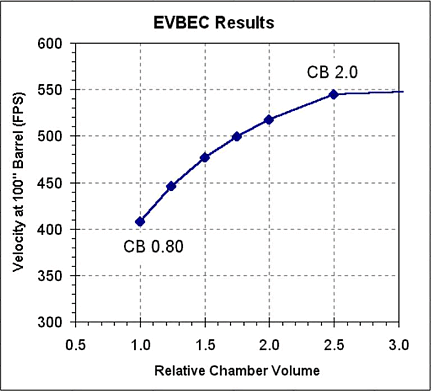# Optimal Combustion Chamber Volume for a Fixed Barrel Size

Combustion spud gunners are always trying to get the best performance out of their guns. One of the most important aspects of the design of a combustion spud gun is the ratio of the size of the combustion chamber to the size of the barrel. In general, for a particular chamber volume there is an optimal barrel volume. The relationship between chamber volume and the barrel volume was examined in the elegant studies performed by Burnt Latke. Latke examined the affect on muzzle velocity of varying the length of the barrel on a fixed size chamber. Latke's data provides the best evidence that the chamber volume divided by the barrel volume (the CB ratio), should be about 0.8 for best performance. For a fixed chamber size the optimal barrel size maximizes both the muzzle velocity and the efficiency of the gun.

Latke's studies not only identified the optimal CB ratio, they also provided a measure of how much a gun's performance will change with non-optimal barrel lengths. In addition, Latke's data provided one of the few measurements of the reproducibility of a well built combustion spud gun. (See for example the SpudWiki on CB ratios.)

Latke's studies show that the though the optimal CB ratio is about 0.8, the affect of small changes in CB near the optimal CB are fairly small. CB ratios in the range of about 0.6 to 1.0 tend to give the same muzzle velocities for a fixed size chamber.

There is another question that has fueled many discussions (and flame wars) on the spud gun forums, namely;

For a fixed size barrel, what CB ratio
maximizes the muzzle velocity?

This question is, in a sense, the opposite of the question Latke's studies were designed to answer. In Latke's studies the chamber volume is held constant and the barrel volume (length) was varied. What would be the results of a study in which the barrel was held constant and the chamber volume varied? Would one obtain the same results as the Latke barrel studies, that is, the optimal CB ratio is ~0.8? Or, does a larger chamber increase the performance for a fixed barrel length? If a larger chamber increases the performance of the barrel is there a limit to how large the chamber can be?

A study of the affect of changing the chamber volume on a fixed length barrel would require that multiple chambers be constructed and tested with the same barrel. Because of the large amount of work required for that study it has not been done.

On this page, I will attempt to calculate the affect on the muzzle velocity of changing the chamber volume on a fixed geometry barrel.

## Mathematical Scaling of The Latke Data to Different SIze Guns

I will use Latke's CB ratio data for his L1 gun firing spuds from a 1.5" diameter (nominal) barrel as the base data set.

 Burnt Latke C:B Study: Optimal barrel length for a fixed chamber size Data from http://www.burntlatke.com/15cb.html Ignition: Dual gap, 65K volts Ammunition: Russets Fuel: Stoichiometric metered propane Oxidizer: Air Chamber fan: 80mm, 12V Ambient temp: 65F Atmospheric pressure: 14.7 psia Chamber Vol.: 158.66 ci (2600cc), 4" Sched. 40 PVC Barrel: 1.5" (ID=1.590") Sched. 40 PVC 12 different barrel lengths 56 total firings (4 to 6 shots per barrel length)

## Calculations

For each barrel in the data set we will;

1. Scale the chamber to a larger volume
2. Scale the energy in the chamber for the larger chamber volume
3. Scale the barrel to maintain the CB ratio for each of Latke's data points
4. Scale the muzzle velocity for the larger chamber and barrel

### Scale the Chamber

To scale the chamber we will simply take Latke's chamber volume (160in3) and multiply by factors of 1.25, 1.5 and 1.75.

### Scale the Energy

To scale the energy in the chamber we will assume that the energy simply scales as the volume of the chamber. Increasing the chamber volume by a factor 50% results in 50% more energy in the chamber. We will assume that the efficiency of the combustion process is the same for all chamber sizes.

### Scale the Barrel

Since we have scaled the chamber we will also scale the volume of the barrel. To keep things consistent we will scale the volume of the barrel by just scaling it's length. We will scale each of Latke's 12 barrel lengths.

### Scale the Muzzle Velocity

Since the chamber volume and barrel length of been scaled we also need to scale the muzzle velocity. This is the most problematic part of the scaling. We are assuming the the overall efficiency of the gun does not change on scaling. Combustion spud guns are typically about 10% efficient, that is, about 10% of the energy available in the chamber actually ends up as kinetic energy of the spud. So, if the efficiency is constant and the chemical energy in the chamber is proportional to the chamber volume then we can say that;

Chemical energy in the chamber
is proportional to
the volume of the chamber
which is proportional to
the kinetic energy of the spud.

The relationship between the kinetic energy (KE) of the spud and the spud's velocity is of course just;

KE =(1/2)mv 2

Where m is the mass of the spud and v is the muzzle velocity of the spud. Since the mass of the spud is being held constant, we can write;

V2 / V1 = v22 / v12

Where V2 is the scaled chamber volume, V1 is the Latke chamber volume, v1 is the Latke muzzle velocity and v2 is the calculated velocity for the scaled gun. Since we actually want the muzzle velocity we take the square root of both sides of the equation and rearrange it to;

v2 = (v1)SQRT(V2 / V1)

In other words, we scale the velocity by the square root of the ratio of the chamber volumes.

As an example, consider the Latke data for the 100 inch barrel (for which the CB is 0.8). The chamber volume will be scaled by 1.25. The barrel length (and therefore it's volume) is also scaled by 1.25 to a length of 125 inches. The muzzle velocity calculated for this new chamber and barrel combination is;

v2 = (514FPS)SQRT(1.25/1.00) = 575 FPS

## Results

The table below lists the original Latke data (in the first three columns) as well as the calculated values for scaling the chamber volume by factors of 1.25, 1.5 and 1.75 (Rel. Chamber Vol.). For the first row of the 1.25 relative chamber volume gun I have included the math used to calculate the values and color coded the values used. Since we are interested in how changing the chamber volume affects the muzzle velocity for a fixed size (length) barrel, I have highlighted the results for the 100 inch barrel for each of the guns.

 Relative Chamber Vol. = 1.00 Rel. Chamber Vol. = 1.25 Rel. Chamber Vol. = 1.5 Rel. Chamber Vol. = 1.75 Barrel   Length (inch) C:B   Ratio Average Velocity (FPS) Barrel Length (inch) C:B Ratio Calculated Velocity (FPS) Barrel Length (inch) C:B Ratio Calculated Velocity (FPS) Barrel Length (inch) C:B Ratio Calculated Velocity (FPS) 265.0 0.30 334 (265)(1.25) = 331.3 0.30 (334)sqrt(1.25) = 373 397.5 0.30 409 463.8 0.30 442 200.0 0.40 430 250.0 0.40 481 300.0 0.40 527 350.0 0.40 569 160.0 0.50 513 200.0 0.50 574 240.0 0.5 629 280.0 0.50 679 132.0 0.60 521 165.0 0.60 582 198.0 0.60 638 231.0 0.60 689 114.0 0.70 519 142.5 0.70 581 171.0 0.70 636 199.5 0.70 687 100.0 0.80 514 125.0 0.80 575 150.0 0.80 629 175.0 0.80 680 89.0 0.90 510 111.3 0.90 570 133.5 0.90 625 155.8 0.90 675 80.0 1.00 497 100.0 1.00 556 120.0 1.00 609 140.0 1.00 658 72.5 1.10 489 90.6 1.10 546 108.8 1.10 599 126.9 1.10 647 66.5 1.20 485 83.1 1.20 543 99.8 1.20 594 116.4 1.20 642 61.5 1.30 442 76.9 1.30 494 92.3 1.30 541 107.6 1.30 585 57.0 1.40 343 71.3 1.40 383 85.5 1.40 420 99.8 1.40 453

A graph of the data is shown below.In this graph the black curve is the original Latke data; variable barrel length on a fixed size chamber. The thick vertical green line marks the constant 100" long barrel, this is the 0.8 CB barrel for Latke's chamber.

The graph for the calculated gun with a 25% larger chamber (red line) shows that the muzzle velocity is predicted to increase with the larger chamber, from 514 FPS to 556 FPS. For the 50% larger chamber (blue line) the muzzle velocity also increases, from 514 FPS to 594 FPS.

Interestingly, for the calculated gun with a 75% larger chamber the muzzle velocity is predicted to drop off substantially, from 514 FPS to 453 FPS for the constant barrel length. The predicted muzzle velocity is not only less than that for the next smaller chamber size, it is also less than the velocity of the original chamber size.

For each of the chamber sizes we can also calculate the CB ratio for the 100 inch long barrel. A graph of the muzzle velocity as a function of the CB ratio is given below.As you can see, the scaling model predicts that, for a fixed size barrel, the maximum muzzle velocity is obtained with a CB ratio of about 1.2. If the CB ratio is increased (the chamber is made bigger) beyond 1.2 then the muzzle velocity drops off dramatically. It looks like the performance would be pretty poor at a CB of 1.5.

This result is fundamentally different than the Latke result. In the Latke studies the performance and efficiency of the chamber is being optimized by changing the barrel length. In this modeling study we are maximizing performance without regard to efficiency. Indeed, the gun scaled by 1.5 (which gives a CB of 1.2 on with the 100" barrel) is less efficient than that chamber would be on a longer barrel.

 Gun Change CB Ratio Rel. Chamb. Vol. Muzzle Velocity (FPS) Relative Velocity Chamber Length (inch) Barrel Length (inch) Total Gun Length (ft) Efficiency Rel. KE Original Latke L1 with CB 0.8 Barrel 0.8 1 514 1 14 100 9.5 7.7% 1 Increase chamber volume by 50% 1.2 1.5 594 1.16 21 10.1 6.9% 1.34 Increase barrel length by 50% to match the 50% larger chamber 1.2 630 1.23 150 14.2 7.7% 1.50

It appears that a 50% change in the length of the chamber, which is a small change in the total length of the gun (7 inches total out of 9.5 feet), increases the muzzle velocity by 16% and the kinetic energy by 34%. To get the full benefit of the larger chamber size requires a significant increase in the total length of the barrel and gun (4.2 feet longer) but the much larger length gun only increases the muzzle velocity by an additional 7% and the KE by 16%.

## Modeling Conclusion

This mathematical model suggests that there is indeed an optimal CB that maximizes muzzle velocity for a fixed length barrel. The optimal CB appears to be about 1.2. The velocity does drop off with an oversized chamber. Furthermore, the drop off occurs at CB's greater than about 1.3 and the drop in muzzle velocity is substantial.

## So, Lets Design a Gun Using a CB of 1.2

The first thing we need to decide is how big the gun is going to be. Is it a "mini" that can be held and fired with one hand? Rifle size that can be fired from the shoulder or hip, or a cannon that needs a carriage? Lets say we are going for a gun that will be fired from the hip, so, a practical total length of about 5 feet seems right. Next, we need to decide the diameters of the chamber and barrel. My local hardware store only has pressure rated PVC up to 3" diameter so that is what we'll use for the chamber. What about the barrel? A 2" ID barrel is common for firing spuds but spuds big enough to seal a 2" barrel are not all that common and tend to be expensive. So, we'll use a 1.5" ID barrel instead, most any spud will fit and we can fire other things like crab apples, zuchini, etc.

That gives a 5 foot gun made with a 3" ID chamber and a 1.5" ID barrel. We'll use a CB of 1.2 to maximize the muzzle velocity (at the expensive of efficency). With these parameters we can write out all the equations and solve for the chamber and barrel lengths that will give the 1.2 CB and an overall length (LT) of 5 feet. The CB ratio is given by;

 Volume Chamber π(rc2)Lc (rc2)Lc = = = CB ( 1 ) Volume Barrel π(rb2)Lb (rb2)Lb

Where Lc is the length of the chamber and Lb is the length of the barrel, rc is the radius of the chamber and rb is the radius of the barrel. The length of the chamber and the barrel are related to our total gun length by;

 LT = Lc + Lb ( 2 )

We can solve equation 2 for Lc ;

 Lc = LT - Lb ( 3 )

and then replace Lc  in equation 1 with LT - Lb from equation 3;

 Volume Chamber (rc2)(LT - Lb) = = CB ( 4 ) Volume Barrel (rb2)Lb

Solving euation 4 for  Lb;

 (rc2)(LT - Lb) = CB(rb2)(Lb) (rc2)(LT) - (rc2)(Lb) = CB(rb2)(Lb) (rc2)(LT) = CB(rb2)(Lb) + (rc2)(Lb) (rc2)(LT) = (Lb)((CB)rb2 + rc2) (rc2 )(LT) Lb = ( 5 ) (CB)(rb2) + rc2

The ID of 3" Sch. 40 PVC is 3.04" so rc=1.52", for the 1.5" barrel the ID is 1.59" so rb=0.795" and using our targeted total length of 5 feet (60") and CB=1.2 gives;

 (1.522in2)(60in) Lb = (1.2)(0.7952in2) + 1.522in2 Lb = 45.2in

Plugging this value for Lb into equation 2 we can solve for the length of the chamber;

 Lc = LT - Lb Lc = 60in - 45.2in Lc = 14.8in

This gives us a gun with CB 1.2 and an overall length of 5 feet. The chamber volume is 107.4in3 and the barrel volume is 89.8in3. We can use Latke's muzzle velocity value for his 1.2 CB gun to estimate the muzzle velocity of this gun;

 estimated muzzle velocity = Sqrt(this chamber volume/Latke's chamber volume) x Latke's muzzle velocity

 estimated muzzle velocity = Sqrt(107.4/160) x 485 FPS = 397 FPS

What would happen if instead of a 5 foot gun with CB=1.2 we designed it to a CB of 0.8? Using equations 5 and 2, and a CB of 0.8, we get a chamber length of 11" and a barrel length of 49". Scaling the Latke velocity for an 0.8 CB we get a predicted muzzle velocity of 367 FPS.

Of course, YMMV.

## Calculation using EVBEC_Live_1.5

EVBEC is a modelling program for combustion spud guns. EVBEC uses the measured performance of a couple of guns and a mathematic model to predict the performance of a gun with user defined parameters. I have used EVBEC to predict the performance of Latke's L1 gun and then examined the prediction as the volume of the chamber is increases but the barrel held constant. A screen of shot of EVBEC showing the initial parameters is shown below. The 2.12 oz (60g) projectile mass is an estimate of what a spud would weigh when cut to a 1.5" barrel. The EVBEC predictions are sensitive to the projectile mass (as they should be).The table below lists the chamber volumes and the predicted muzzle velocities calculated by EVBEC.

 Chamber Volume (in3) Chamber Vol. Ratio Barrel Length (in) CB Ratio Velocity (FPS) Rel. Velocity 160 1.00 100 0.80 408 1.00 200 1.24 100 1.00 446 1.09 240 1.51 100 1.21 476 1.17 280 1.75 100 1.41 499 1.22 320 2.00 100 1.61 517 1.27 400 2.50 100 2.01 545 1.34 3200 20 100 16.1 632 1.55

Below is a graph of the EVBEC predicted muzzle velocities as a function of the chamber size.EVBEC predicts that for a fixed barrel size (and ammo) the muzzle velocity always increases as the chamber volume is increased. This is what happens in pneumatic guns and seems to be incorrect for a combustion gun. Grossly oversized chambers decrease the performance of combustion guns.

The EVBEC predicted muzzle velocity for the starting gun is only 408 FPS versus Latke's measured velocity of 514 FPS. It is possible that some of the difference between the Latke results and EVBEC is due to my guess for the spud's mass, though 2.12 oz (60g) seems like a reasonable estimate for half a spud in a 1.6" ID barrel.

Copyright ©2008 J. Sluka
Last Modified:  18 January 2008
Send me an email